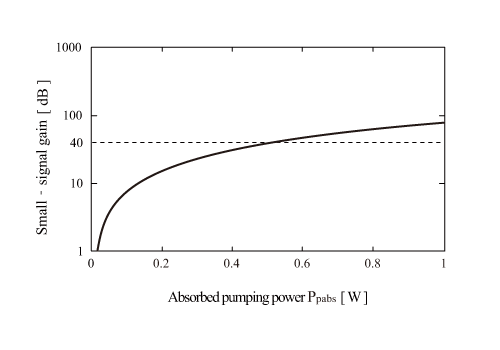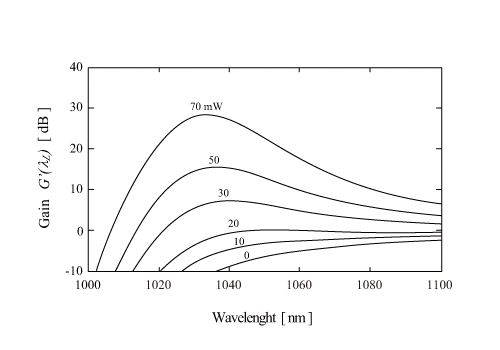In order to analytically solve Pp(Z),  we employ this equation and a following equation,

where ηq is a pumping quantum efficiency, ~1 for Yb3+. The two equations give a following equation,

here Pp(0) is  excitation power at the input edge, and Ppsat is a pumping saturation power.

Pp(z) is a calculated from Eq. 2. In general, Eq. 2 must be numerically solved for the calculation of absorption pumping power by optical fiber length. Provided Pp(0) << Ppsat, Eq. 2 is approximated as follows.

Single pass gain for optical fiber length is expressed by a following equation.

where αL is a damping constant for laser signal light.

The expression of small-signal gain is simplified by Eq. 1. Ppabs, a pumping power, that is absorbed by Yb fiber, is obtained from Eq. 1.

In summary, the small-signal gain, G, is obtained as follows.

The second, and third term explains a reabsorption loss, and a propagation loss of fiber core. When the small-signal gain, G, is converted to dB expression, fiber gain is obtained.

### Clad pumping Yb fiber amplifier

We consider a clad pumping Yb fiber amplifier. Provided the core diameter =10 µm, h = 6.63 x 10-34 Js, τf = 1ms, λp = 975 nm, λL = 1064 nm, σeL) ~2.82×10-25m2, f1 = 0, f2 = 1, σeL)~0, and the propagation loss αL ~ 0 (under the assumption of four states in long wavelength range), the gain characteristics against absorption pumping power is obtained as shown in Fig. 1. The practical small-signal gain is 30~40 dB owing to a parasitic oscillation and and ASE in the optical fiber.Fig. 1 Dependency of gain on pumping power of clad-pumped Yb fiber amplifier

### Core direct pumping Yb fiber amplifier

We consider a core pumping Yb fiber amplifier. Provided the core diameter = 6 µm, τf = 1 ms, ηq = 1, λp = 975 nm, N = 9.5×1025m-3, l = 1 m, and σL ~ 0, the gain characteristics against signal wavelength, G'(λL), is obtained as shown in Fig. 2. The pumping absorption power, Ppabs, is changed between 0 and 70 mW. For the larger pumping power, the peak wavelength of output light is red-shifted, because the practical small-signal gain is 30~40 dB.Fig. 2 Core direct pumping Yb fiber amplifier